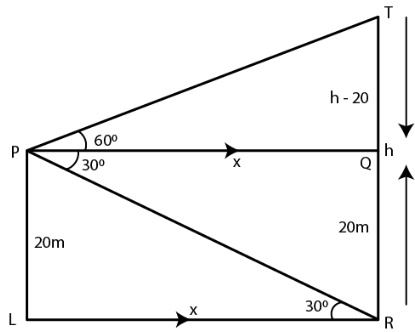Guru

# A vertical pole and a vertical tower are on the same level ground. From the top of the pole the angle of elevation of the top of the tower is 60 and the angle of depression of the foot of the tower is 30. Find the height of the tower if the height of the pole is 20 m.

• 1

sir this is the important  question from the book -ML aggarwal( avichal publication) class 10th , chapter20 , heights and distances
A vertical pole and a vertical tower are on the same level ground.
From the top of the pole the angle of elevation of the top of the tower is 60
and the angle of depression of the foot of the tower is 30.
Find the height of the tower if the height of the pole is 20 m.

question no 34 , heights and distances , ICSE board, ML Aggarwal

Share

1. Consider TR as the tower

PL as the pole on the same level

Ground PL = 20 m

From the point P construct PQ parallel to LR

∠TPQ = 600 and ∠QPR = 300

Here

∠PRL = ∠QPR = 300 which are the alternate angles

Take LR = x and TR = h

TQ = TR – QR = (h – 20) mIn right triangle PRL

tan θ = PL/LR

Substituting the values

tan 300 = 20/x

So we get

1/√3 = 20/x

x = 20√3 m

In right triangle PQT

tan 600 = TQ/PQ

Substituting the values

√3 = (h – 20)/ x

√3 = (h – 20)/ 20√3

By cross multiplication

20√3 × √3 = h – 20

20 × 3 = h – 20

h = 60 + 20 = 80 m

Hence, the height of the tower is 80 m.

• 1